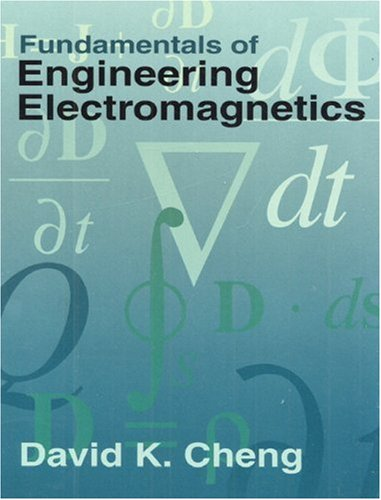### Fundamentals of Engineering Electromagnetics (Addison-Wesley Series in Electrical Engineering)

 Yazar : David K. Cheng Yayınevi : Pearson Education ISBN : 9780201566116 Baskı yılı : 1992 Sayfa sayısı : 495 Ağırlık : 0,95 kg Edisyon : 1 Stok durumu : Tükendi

Fundamental of Engineering Electromagnetics not only presents the fundamentals of electromagnetism in a concise and logical manner, but also includes a variety of interesting and important applications. While adapted from his popular and more extensive work, Field and Wave Electromagnetics, this text incorporates a number of innovative pedagogical features. Each chapter begins with an overview which serves to offer qualitative guidance to the subject matter and motivate the student. Review questions and worked examples throughout each chapter reinforce the students understanding of the material. Remarks boxes following the review questions and margin notes throughout the book serve as additional pedagogical aids.

1. The Electromagnetic Model.

Overview. The electromagnetic model. SI units and universal constants. Summary.

2. Vector Analysis.

Overview. Vector addition and subtraction. Vector multiplication. Orthogonal coordinate systems. Gradient of a scalar field. Divergence of a vector field. Divergence theorem. Curl of a vector field. Stokes theorem. Two null identities. Field classification and Helmholtzs theorem. Summary. Problems.

3. Static Electric Fields.

Overview. Fundamental postulates of electrostatics in free space. Coulombs law. Gausss law and applications. Electric potential. Material media in static electric field. Electric flux density and dielectric constant. Boundary conditions for electrostatic fields. Capacitances and capacitors. Electrostatic energy and forces. Solution of electrostatic boundary-value problems. Summary. Problems.

Overview. Current density and Ohms law. Equation of continuity and Kirchoffs current law. Power dissipation and Joules law. Governing equations for steady current density. Resistance calculations. Summary. Problems.

5. Static Magnetic Fields.

Overview. Fundamental postulates of magnetostatics in free space. Vector magnetic potential. The Biot-Savart law and applications. The magnetic dipole. Magnetization and equivalent current densities. Magnetic field intensity and relative permeability. Behavior of magnetic materials. Boundary conditions for magnetostatic fields. Inductances and inductors. Magnetic energy. Magnetic forces and torques. Summary. Problems.

6. Time-Varying Fields and Maxwells Equations.

Overview. Faradays law of electromagnetic induction. Maxwells equations. Potential functions. Time-harmonic fields. Summary. Problems.

7. Plane Electromagnetic Waves.

Overview. Plane waves in lossless media. Plane waves in lossy media. Group velocity. Flow of electromagentic power and the poynting vector. Normal incidence of plane waves at plane boundaries. Oblique incidence of plane waves at plane boundaries. Summary. Problems.

8. Transmission Lines.

Overview. General transmission-line equations. Transmission-line parameters. Wave characteristics on an infinite transmission line. Wave characteristics on finite transmission lines. The Smith chart. Transmission-line impedance matching. Summary. Problems.

9. Waveguides and Cavity Resonators.

Overview. General wave behaviors along uniform guiding structures. Rectrangular waveguides. Other waveguide types. Cavity resonators. Summary. Problems.

10. Antennas and Antenna Arrays.

Overview. The elemental electric dipole. Antenna patterns and directivity. Thin linear antennas. Antenna arrays. Effective area and backscatter cross section. Friis transmission formula and radar equation. Summary. Problems.

Appendix A: Symbols and Units.

Appendix B: Some Useful Material Constants.

Bibliography.